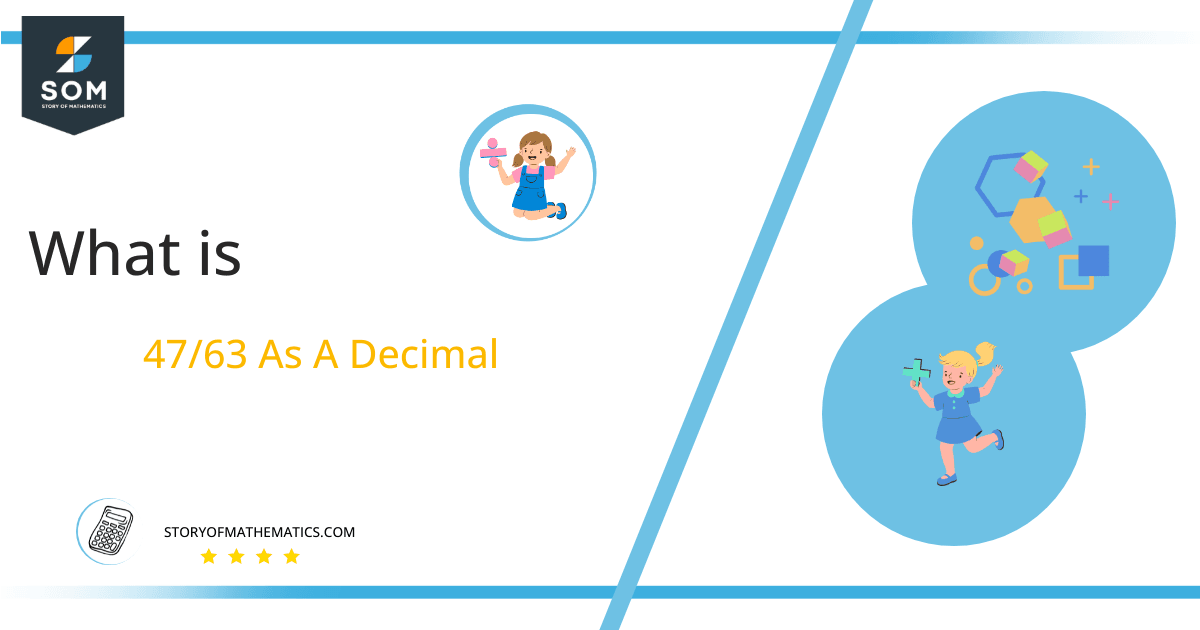# What Is 47/63 as a Decimal + Solution With Free Steps

The fraction 47/63 as a decimal is equal to 0.746.

One of the most essential mathematical operations is division. There are numerous ways to perform the division, including long division. Typically, the division is expressed in the fractional form a/b or decimal form.Here, we are more interested in the division types that result in a Decimal value, as this can be expressed as a Fraction. We see fractions as a way of showing two numbers having the operation of Division between them that result in a value that lies between two Integers.

Now, we introduce the method used to solve said fraction to decimal conversion, called Long Division, which we will discuss in detail moving forward. So, let’s go through the Solution of fraction 47/63.

## Solution

First, we convert the fraction components, i.e., the numerator and the denominator, and transform them into the division constituents, i.e., the Dividend and the Divisor, respectively.

This can be done as follows:

Dividend = 47

Divisor = 63

Now, we introduce the most important quantity in our division process: the Quotient. The value represents the Solution to our division and can be expressed as having the following relationship with the Division constituents:

Quotient = Dividend $\div$ Divisor = 47 $\div$ 63

This is when we go through the Long Division solution to our problem. The Long Division process is shown below in Figure 1:Figure 1

## 47/63 Long Division Method

We start solving a problem using the Long Division Method by first taking apart the division’s components and comparing them. As we have 47 and 63, we can see how 47 is Smaller than 63, and to solve this division, we require that 47 be Bigger than 63.

This is done by multiplying the dividend by 10 and checking whether it is bigger than the divisor or not. If so, we calculate the Multiple of the divisor closest to the dividend and subtract it from the Dividend. This produces the Remainder, which we then use as the dividend later.

Now, we begin solving for our dividend 47, which after getting multiplied by 10 becomes 470.

We take this 470 and divide it by 63; this can be done as follows:

470 $\div$ 63 $\approx$ 7

Where:

63 x 7 = 441

This will lead to the generation of a Remainder equal to 470 – 441 = 29. Now this means we have to repeat the process by Converting the 29 into 290 and solving for that:

290 $\div$ 63 $\approx$ 4

Where:

63 x 4 = 252

This, therefore, produces another Remainder which is equal to 290 – 252 = 38. Now we must solve this problem to Third Decimal Place for accuracy, so we repeat the process with dividend 380.

380 $\div$ 63 $\approx$ 6

Where:

63 x 6 = 378

Finally, we have a Quotient generated after combining the three pieces of it as 0.746=z, with a Remainder equal to 2.Images/mathematical drawings are created with GeoGebra.